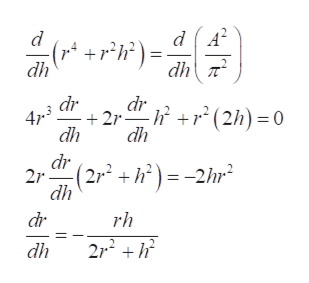# The lateral surface area of a cone of radius r and height h isA=(pi)(r)[r^2+h^2]^(1/2)Find an expression for dr/dh

Question
67 views

The lateral surface area of a cone of radius r and height h is

A=(pi)(r)[r^2+h^2]^(1/2)

Find an expression for dr/dh

check_circle

Step 1

Refer to the question, differentiate the provided lateral surface area with respect h as,

Step 2

Then transform the lateral surface area of the cone in terms of r as,

Step 3

Now  different...help_outlineImage TranscriptionclosedA d dh dr dr +2r dh -+ (2h) 0 47.3 dh dr 2r dh (2r2 +h)= -2hr2 dr rh 2r2 dh fullscreen

### Want to see the full answer?

See Solution

#### Want to see this answer and more?

Solutions are written by subject experts who are available 24/7. Questions are typically answered within 1 hour.*

See Solution
*Response times may vary by subject and question.
Tagged in
MathCalculus

### Derivative